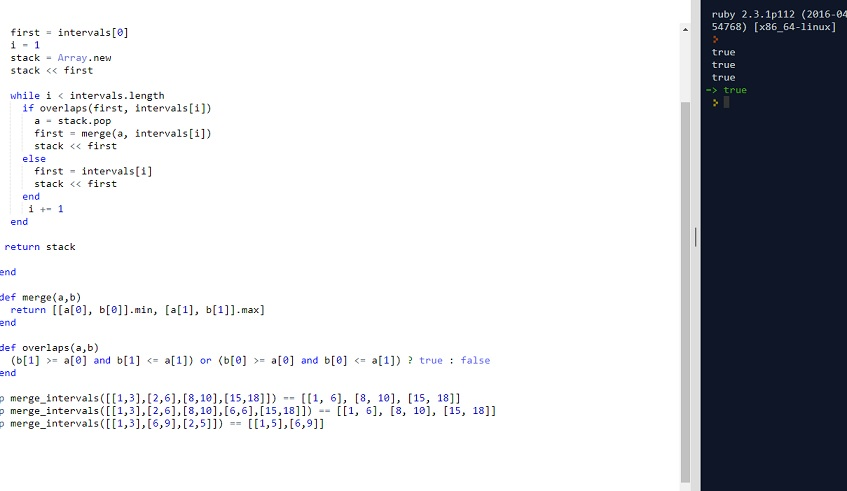# CLEAN CODE: MERGE INTERVALS | ARRAY

By: Saurav

2018-02-06 00:45:00 UTC

Given a set of non-overlapping intervals, insert a new interval into the intervals (merge if necessary).

You may assume that the intervals were initially sorted according to their start times.

Example 1:

Given intervals [1,3],[6,9] insert and merge [2,5] would result in [1,5],[6,9].

Example 2:

Given [1,2],[3,5],[6,7],[8,10],[12,16], insert and merge [4,9] would result in [1,2],[3,10],[12,16].

This is because the new interval [4,9] overlaps with [3,5],[6,7],[8,10].

Make sure the returned intervals are also sorted.

InterviewBit

There are various ways of solving this problem. One approach can be to use hash and store key and value as number line and presence. But that would take O(n**2) time

A really efficient way would be to use stack.

Algo:
1. Sort the intervals
2. Add the first interval in the stack
3. Starting from the second interval, check if the top interval in the stack overlaps with the present interval
If it does, pop the top one, merge the intervals and add to the stack
If it doesn't, add the second one, update top and continue with third value

Let's start with writing tests first.

```p merge_intervals([[1,3],[2,6],[8,10],[15,18]]) == [[1, 6], [8, 10], [15, 18]]
p merge_intervals([[1,3],[2,6],[8,10],[6,6],[15,18]]) == [[1, 6], [8, 10], [15, 18]]
p merge_intervals([[1,3],[6,9],[2,5]]) == [[1,5],[6,9]]

```

lets see the code:

```def merge_intervals(intervals)
return unless intervals
return [] if intervals.length < 1

intervals.sort!{|a,b| a <=> b}

first = intervals
i = 1
stack = Array.new
stack << first

while i < intervals.length
if overlaps(first, intervals[i])
a = stack.pop
first = merge(a, intervals[i])
stack << first
else
first = intervals[i]
stack << first
end
i += 1
end

return stack

end

def merge(a,b)
return [[a, b].min, [a, b].max]
end

def overlaps(a,b)
(b >= a and b <= a) or (b >= a and b <= a) ? true : false
end
```

All our tests pass as shown below:Looks good to me.

### Let me know what you think! twitter: sprakash24octlinkedin

Owned & Maintained by Saurav Prakash

If you like what you see, you can help me cover server costs or buy me a cup of coffee though donation :)